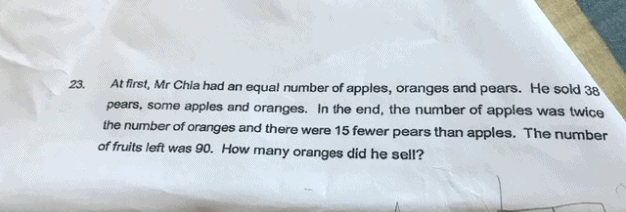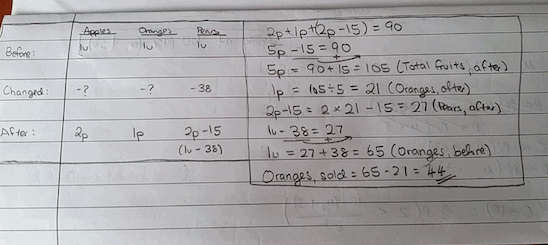# QuestionPls kindly help and assist on p5 math .. Thanks a lot ..Check (to see if the answer is correct)

Apples sold = 65 – 2 x 21 = 23

Oranges sold = 44

Pears sold = 38

Total, after = (65 – 23) + (65 – 44) + (65 – 38) = 42 + 21 + 27 = 90 (Correct -> matches with the no. given in the question)

Hope you have understood my answer! Suma.

0 Replies 1 Like